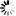# QD (Library)

## Introduction

QD is a package that supports both a double-double datatype with about 32 decimal digits,

The library is written by David H. Bailey et. al from the Lawrence Berkeley National Laboratory (see http://crd.lbl.gov/~dhbailey/mpdist).

In addition to the basic arithmetic operations (add, subtract, multiply, divide, square root), common transcendental functions such as the exponential, logarithm, trigonometric and hyperbolic functions are also included.

## Availability Table

System Versions
iqaluk 2.3.13, 2.3.17
copper 2.3.13, 2.3.17
wobbie 2.3.13, 2.3.17
vdi-centos6 2.3.13, 2.3.17